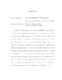## Quasiperiodicity and ChaosIn this work, we investigate a property called multi-chaos'' is which a chaotic set has densely many hyperbolic periodic points of unstable dimension $k$ embedded in it, for at least 2 different values of $k$. We construct a family of maps on the torus having this property. They serve as a paradigm for multi-chaos occurring in higher dimensional systems. One of the factors that leads to this strong form of chaos is the occurrence of a quasiperiodic orbit transverse to an expanding sub-bundle of the tangent bundle. Hence, a key step towards identifying multi-chaos numerically is finding quasiperiodic orbits in high dimensional systems. To analyze quasiperiodic orbits, we develop a method of weighted ergodic averages and prove that these averages have super-polynomial convergence to the Birkhoff average. We also show how this accelerated convergence of the ergodic averages over quasiperiodic trajectories enable us to compute the rotation number, Fourier series and Lyapunov exponents of quasiperiodic orbits with a high degree of precision ($\approx 10^{-30}$).